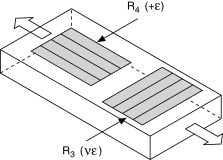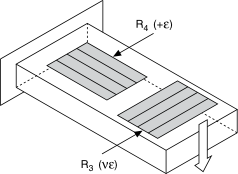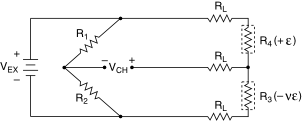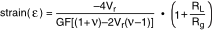# Half-Bridge Type I

## NI-DAQmx 18.6 Help

Edition Date: January 2019
Part Number: 370466AH-01
View Product InfoNI-DAQmx 18.6 HelpNI-DAQmx 19.0 HelpNI-DAQmx 19.1 HelpNI-DAQmx 19.5 Help

The following figure shows how to position strain gage resistors in an axial configuration for the half-bridge type I.The following figure shows how to position strain gage resistors in a bending configuration for the half-bridge type I.Half-bridge type I strain gage configurations have the following characteristics:
• Two active strain gage elements, one mounted in the direction of axial strain and the other acting as a Poisson gage and mounted transverse, or perpendicular, to the principal axis of strain.
• Completion resistors which provide half-bridge completion.
• Sensitivity to both axial and bending strain.
• Compensation for temperature.
• Compensation for the aggregate effect on the principle strain measurement due to the Poisson's ratio of the material.
• Sensitivity at 1000 µε is ~ 0.65 mVout/ VEX input
Related concepts

## Half-Bridge Type I Circuit DiagramThe following symbols apply to the circuit diagram:
• R1 is the half-bridge completion resistor.
• R2 is the half-bridge completion resistor.
• R3 is the active strain gage element measuring compression due to the Poisson effect (–ε).
• R4 is the active strain gage element measuring tensile strain (+ε).
• VEX is the excitation voltage.
• RL is the lead resistance.
• VCH is the measured voltage.

The following equation converts voltage ratios to strain units for half-bridge type I configurations.where Vr is the voltage ratio that virtual channels use in the voltage-to-strain conversion equation, GF is the gage factor, v is the Poisson's ratio, RL is the lead resistance, and Rg is the nominal gage resistance.